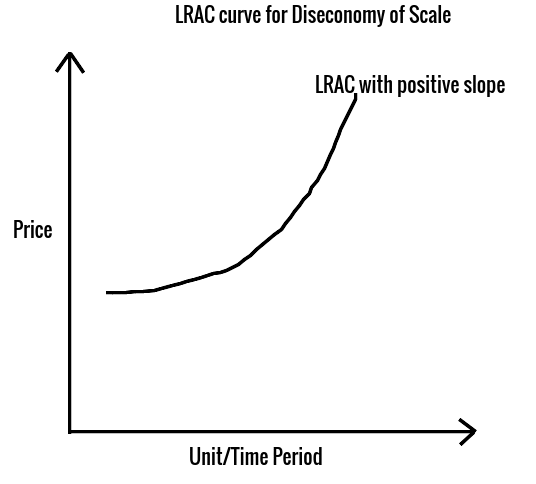# Understanding Economies and Diseconomies of Scale

Scaling up refers to a firm increasing all of its inputs in order to increase its level of output. On the other hand, Scaling down refers to a firm decreasing all of its inputs in order to decrease its level of output.
1. Economies of Scale means that with rising levels of output, cost per unit of production decreases. This is because as the level of production rises, Fixed cost per unit of production decreases, so Long Range Average Total Cost decreases.
2. Diseconomies of Scale means that with rising levels of output, cost per unit of production increases. This happens when the Long Range Average Total Cost increases with the rise in output.

## Time Frame to decide Scale

• The firm usually selects an operating size or scale that maximizes profit over any time frame. There are two-time frames: Short Run and Long Run, depending upon the ability of the firm to adjust the quantities of the fixed resources it uses.
• The short-run is the time period during which at least one of the factors of production, such as technology, physical capital, and plant size, is fixed. While the long run is defined as the time period during which all factors of production are variable.
• The long-run is referred to as the “planning horizon” in which the firm can decide upon the optimal operating size (Scale) that maximizes profit over time. The firm is always operating in the short run but planning in the long run.
• The time period of the short-run and long-run varies from firm to firm. For some firms like ice cream production units, the short term will be of say 1 year, while for cement manufacturing firms, the short term will be of 4-5 years. The only thing that matters is that in the long term all the factors of production like labor, capital, etc. should be variable.
• The short-run total cost (STC) will include all the inputs like labor and capital that the firm uses to produce output. Initially, the short-run cost (STC) increases with a rise in output but at a slower rate because of specialization and division of labor. Later it increases with increasing rate. (Law of Diminishing Marginal Return)

## Economies & Diseconomies of Scale

### Economics of Scale

• Economies of Scale means that with rising levels of output, cost per unit of production decreases. This is because as the level of production rises, Long Range Average Total Cost (LRAC) decreases. The LRAC curve has a negative slope as shown below:• As the firm grows in size, economies of scale and a lower Average Total Cost (ATC) can result from the following factors:
• An increase in returns due to a production process that allows for an increase in output that is larger than the increase in the cost of inputs.
• Division of labor and management in large firms leads to specialization and hence lower cost.
• Firms are able to afford expensive but efficient equipment giving a technological edge.
• Reducing waste and lowering costs through marketable byproducts, less energy consumption, and enhanced quality control.
• Making better use of market information and knowledge for more effective managerial decision making.
• Obtaining discounted prices on resources when buying in larger quantities.

### Diseconomies of Scale

• Diseconomies of Scale means that with rising levels of output, cost per unit of production increases. This happens when the Long Range Average Total Cost increases with a rise in output. The LRAC curve has a positive slope as shown below:• The factors that can lead to diseconomies of scale, inefficiencies, and rising costs when a firm increases in size include the following:
1. A decrease in returns to scale due to a production process that leads to increases in the cost of inputs that are larger than the increase in output.
2. Poor management of the firm due to its large size or geographical spreading.
3. Overlapping and duplication of business functions.
4. Higher resource prices because of supply constraints when buying inputs in large quantities.